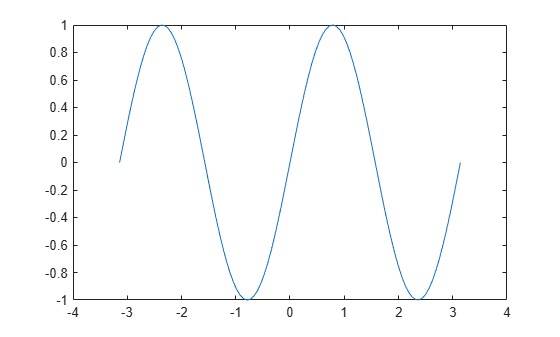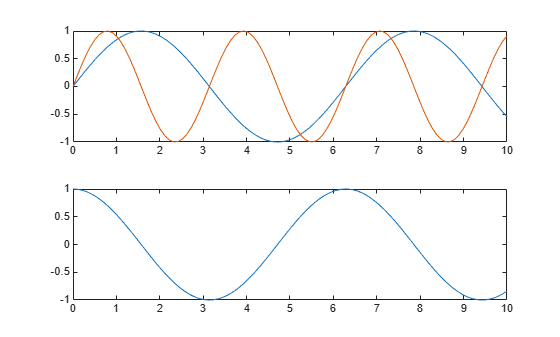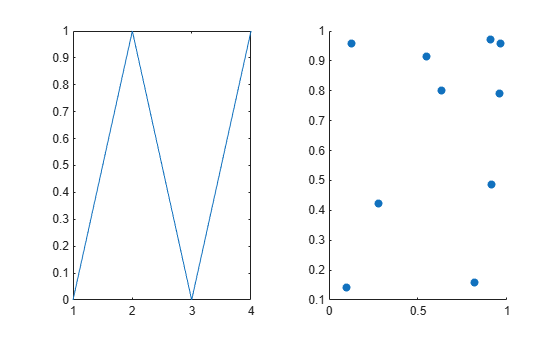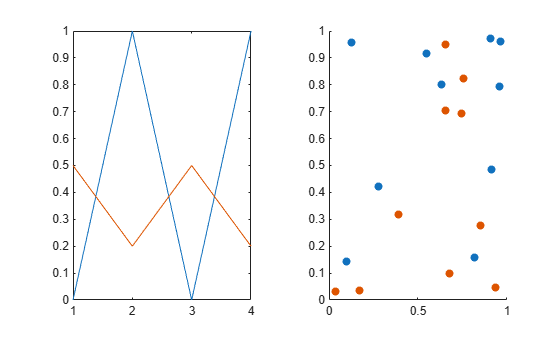# hold

Retain current plot when adding new plots

## Syntax

``hold on``
``hold off``
``hold all``
``hold``
``hold(ax,___)``

## Description

example

````hold on` retains plots in the current axes so that new plots added to the axes do not delete existing plots. New plots use the next colors and line styles based on the `ColorOrder` and `LineStyleOrder` properties of the axes. MATLAB® adjusts axes limits, tick marks, and tick labels to display the full range of data. If axes do not exist, then the `hold` command creates them.```

example

````hold off` sets the hold state to off so that new plots added to the axes clear existing plots and reset all axes properties. The next plot added to the axes uses the first color and line style based on the `ColorOrder` and `LineStyleOrder` properties of the axes. This option is the default behavior.```
````hold all` is the same as `hold on`. This syntax will be removed in a future release. Use ```hold on``` instead.```
````hold` toggles the hold state between on and off.```

example

````hold(ax,___)` sets the hold state for the axes specified by `ax` instead of the current axes. Specify `ax` before all other arguments in any of the previous syntaxes. Use single quotes around the `'on'` and `'off'` inputs, such as `hold(ax,'on')`.```

## Examples

collapse all

Create a line plot. Use `hold on` to add a second line plot without deleting the existing line plot. The new plot uses the next color and line style based on the `ColorOrder` and `LineStyleOrder` properties of the axes. Then reset the hold state to off.

```x = linspace(-pi,pi); y1 = sin(x); plot(x,y1) hold on y2 = cos(x); plot(x,y2) hold off```When the hold state is off, new plots delete existing plots. New plots start from the beginning of the color order and line style order.

```y3 = sin(2*x); plot(x,y3)```Starting in R2019b, you can display a tiling of plots using the `tiledlayout` and `nexttile` functions. Call the `tiledlayout` function to create a 2-by-1 tiled chart layout. Call the `nexttile` function to create the axes objects `ax1` and `ax2`. Plot a sine wave plot in each axes.

```x = linspace(0,10); y1 = sin(x); y2 = cos(x); tiledlayout(2,1) % Top plot ax1 = nexttile; plot(ax1,x,y1) % Bottom plot ax2 = nexttile; plot(ax2,x,y2)```Add a second sine wave to the top axes.

```hold(ax1,'on') y3 = sin(2*x); plot(ax1,x,y3) hold(ax1,'off')```Create a 1-by-2 tiled chart layout. Call the `nexttile` function to create two axes objects and plot into the axes.

```t = tiledlayout(1,2); ax1 = nexttile; ax2 = nexttile; plot(ax1,[0 1 0 1]) scatter(ax2,rand(1,10),rand(1,10),'filled')```Set the `hold` state for both of the axes to `'on'`. Then display additional data in each plot.

```hold([ax1 ax2],'on') plot(ax1,[.5 .2 .5 .2]) scatter(ax2,rand(1,10),rand(1,10),'filled')```## Input Arguments

collapse all

Target axes, specified as one of the following:

• Any type of axes object: an `Axes`, `PolarAxes`, or `GeographicAxes` object.

• An array of axes objects that belong to the same class. To determine the class, use the `class` function.

If you do not specify the axes, then `hold` sets the hold state for the current axes.

## Tips

• Use the `ishold` function to test the hold state.

## Algorithms

The `hold` function sets these properties:

• `NextPlot` axes property - Sets this property of the associated `Axes`, `PolarAxes`, or `GeographicAxes` object to either `'add'` or `'replace'`.

• `NextPlot` figure property - Sets this property of the `Figure` object to `'add'`.

### Topics

Introduced before R2006a